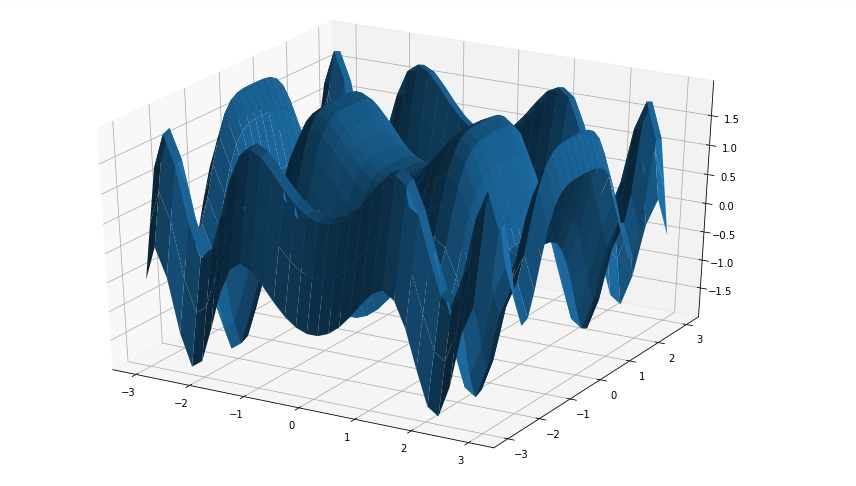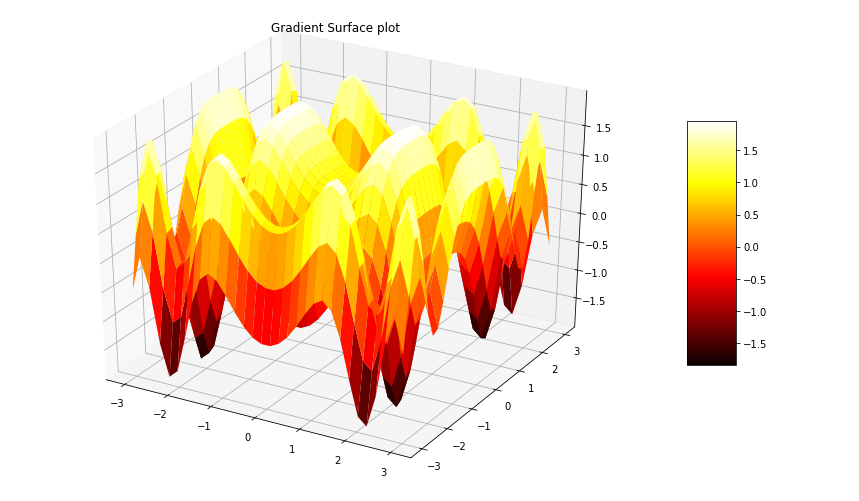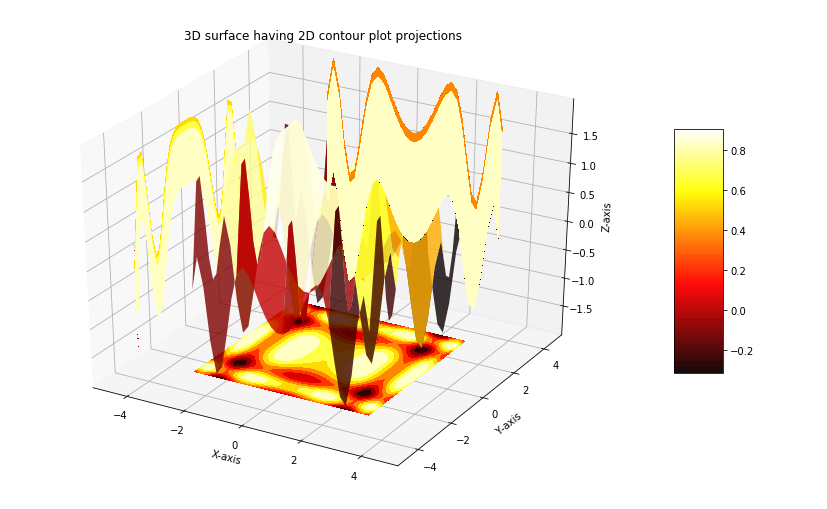GFG App
Open AppBrowser
Continue

# 3D Surface plotting in Python using Matplotlib

A Surface Plot is a representation of three-dimensional dataset. It describes a functional relationship between two independent variables X and Z and a designated dependent variable Y, rather than showing the individual data points. It is a companion plot of the contour plot. It is similar to the wireframe plot, but each face of the wireframe is a filled polygon. This helps to create the topology of the surface which is being visualized.

## Creating 3D surface Plot

The axes3d present in Matplotlib’s mpl_toolkits.mplot3d toolkit provides the necessary functions used to create 3D surface plots.Surface plots are created by using ax.plot_surface() function.
Syntax:

`ax.plot_surface(X, Y, Z)`

where X and Y are 2D array of points of x and y while Z is 2D array of heights.Some more attributes of ax.plot_surface() function are listed below:

Example: Let’s create a 3D surface by using the above function

## Python3

 `# Import libraries` `from` `mpl_toolkits ``import` `mplot3d` `import` `numpy as np` `import` `matplotlib.pyplot as plt`     `# Creating dataset` `x ``=` `np.outer(np.linspace(``-``3``, ``3``, ``32``), np.ones(``32``))` `y ``=` `x.copy().T ``# transpose` `z ``=` `(np.sin(x ``*``*``2``) ``+` `np.cos(y ``*``*``2``) )`   `# Creating figure` `fig ``=` `plt.figure(figsize ``=``(``14``, ``9``))` `ax ``=` `plt.axes(projection ``=``'3d'``)`   `# Creating plot` `ax.plot_surface(x, y, z)`   `# show plot` `plt.show()`

Output:Gradient surface plot is a combination of 3D surface plot with a 2D contour plot. In this plot the 3D surface is colored like 2D contour plot. The parts which are high on the surface contains different color than the parts which are low at the surface.
Syntax:

surf = ax.plot_surface(X, Y, Z, cmap=, linewidth=0, antialiased=False)

The attribute cmap= sets the color of the surface. A color bar can also be added by calling fig.colorbar. The code below create a gradient surface plot:
Example:

## Python3

 `# Import libraries` `from` `mpl_toolkits ``import` `mplot3d` `import` `numpy as np` `import` `matplotlib.pyplot as plt`   `# Creating dataset` `x ``=` `np.outer(np.linspace(``-``3``, ``3``, ``32``), np.ones(``32``))` `y ``=` `x.copy().T ``# transpose` `z ``=` `(np.sin(x ``*``*``2``) ``+` `np.cos(y ``*``*``2``) )`   `# Creating figure` `fig ``=` `plt.figure(figsize ``=``(``14``, ``9``))` `ax ``=` `plt.axes(projection ``=``'3d'``)`   `# Creating color map` `my_cmap ``=` `plt.get_cmap(``'hot'``)`   `# Creating plot` `surf ``=` `ax.plot_surface(x, y, z,` `                       ``cmap ``=` `my_cmap,` `                       ``edgecolor ``=``'none'``)`   `fig.colorbar(surf, ax ``=` `ax,` `             ``shrink ``=` `0.5``, aspect ``=` `5``)`   `ax.set_title(``'Surface plot'``)`   `# show plot` `plt.show()`

Output:## 3D surface Plot having 2D contour plot projections

3D surface plots plotted with Matplotlib can be projected on 2D surfaces. The code below creates a 3D plots and visualizes its projection on 2D contour plot:
Example:

## Python3

 `# Import libraries` `from` `mpl_toolkits ``import` `mplot3d` `import` `numpy as np` `import` `matplotlib.pyplot as plt`   `# Creating dataset` `x ``=` `np.outer(np.linspace(``-``3``, ``3``, ``32``), np.ones(``32``))` `y ``=` `x.copy().T ``# transpose` `z ``=` `(np.sin(x ``*``*``2``) ``+` `np.cos(y ``*``*``2``) )`   `# Creating figure` `fig ``=` `plt.figure(figsize ``=``(``14``, ``9``))` `ax ``=` `plt.axes(projection ``=``'3d'``)`   `# Creating color map` `my_cmap ``=` `plt.get_cmap(``'hot'``)`   `# Creating plot` `surf ``=` `ax.plot_surface(x, y, z, ` `                       ``rstride ``=` `8``,` `                       ``cstride ``=` `8``,` `                       ``alpha ``=` `0.8``,` `                       ``cmap ``=` `my_cmap)` `cset ``=` `ax.contourf(x, y, z,` `                   ``zdir ``=``'z'``,` `                   ``offset ``=` `np.``min``(z),` `                   ``cmap ``=` `my_cmap)` `cset ``=` `ax.contourf(x, y, z,` `                   ``zdir ``=``'x'``,` `                   ``offset ``=``-``5``,` `                   ``cmap ``=` `my_cmap)` `cset ``=` `ax.contourf(x, y, z, ` `                   ``zdir ``=``'y'``,` `                   ``offset ``=` `5``,` `                   ``cmap ``=` `my_cmap)` `fig.colorbar(surf, ax ``=` `ax, ` `             ``shrink ``=` `0.5``,` `             ``aspect ``=` `5``)`   `# Adding labels` `ax.set_xlabel(``'X-axis'``)` `ax.set_xlim(``-``5``, ``5``)` `ax.set_ylabel(``'Y-axis'``)` `ax.set_ylim(``-``5``, ``5``)` `ax.set_zlabel(``'Z-axis'``)` `ax.set_zlim(np.``min``(z), np.``max``(z))` `ax.set_title(``'3D surface having 2D contour plot projections'``)`   `# show plot` `plt.show()`

Output:My Personal Notes arrow_drop_up